## Organic chemistry - some basic principles and techniques Quiz-25

IIT JEE exam which consists of JEE Main and JEE Advanced is one of the most important entrance exams for engineering aspirants. The exam is held for candidates who are aspiring to pursue a career in the field of engineering and technical studies. Chemistry is important because everything you do is chemistry! Even your body is made of chemicals. Chemical reactions occur when you breathe, eat, or just sit there reading. All matter is made of chemicals, so the importance of chemistry is that it's the study of everything..

Q1.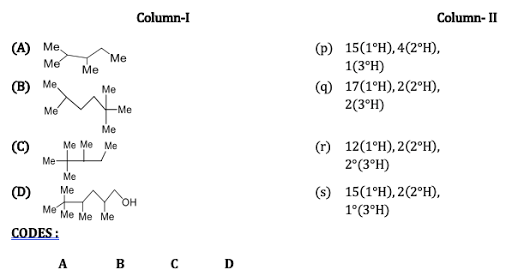•  P s q r
•  r p s q
•  s q r p
•  q r p s
Solution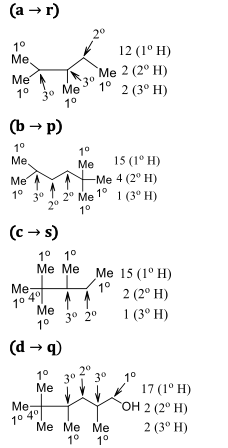Q2.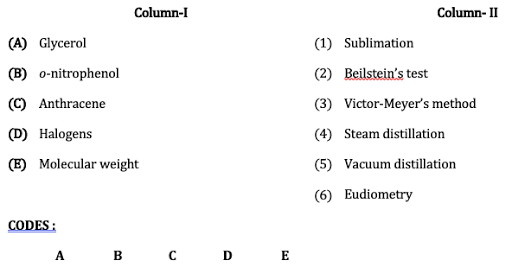•  5 4 1 2 3
•  4 5 1 6 3
•  6 4 1 3 3
•  5 4 6 2 3
Solution

Sublimation conversion of solid directly into gaseous phase. The liquid state does not exist. e.g., naphthalene, anthracene. Beilstein test simple chemical test for halogens. Victor-Meyer’s method Standard laboratory method for determining the molecular weight of a volatile liquid. Steam distillation vaporisation of the volatile constituents of a liquid. It is special type of distillation for a temperature sensitive materials like aromatic natural compounds. e.g., ortho nitrophenol, cinnamaldehyde. Vacuum distillation at reduced pressure. It is generally used in case of high boiling liquids which decompose below their normal boiling points, cannot be purified by distillation at atmospheric pressure e.g., glycerol. Eudiometry it is the process of determining the constituents of a gaseous mixture by eudiometer. It is used for ascertaining the purity of air or amount of O_2 in it.

Q3. In which of the following reactions, the principal group loses its preferences?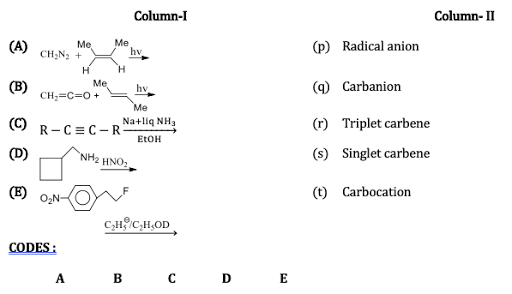•  t s p r p
•  p s r p p
•  t p s r p
•  s r p t p
Solution
(d) (c→p) (d→t) (e→p) (E 1 mechanism)

Q4.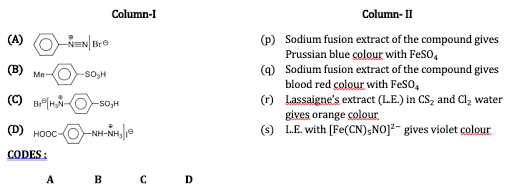>
•  s p r q,r
•  r s q,r p
•  p r q s
•  q,r q s r
Solution
(a r) Diazonium salts do not give positive test for N, because on heating they decompose before combining with Na to form ion, which is a necessary condition for n- test On the other hand, it gives the test for ion (organic layer test) (b s) It contains C and S, so it gives nitroprusside test. , is nitrorpuside ion (c q,r) It contain C and N, so it gives (positive) test for N and the test for (d p) It contains C and N, so it gives positive test for N and also gives test for (but that is not given in the equation)

Q5.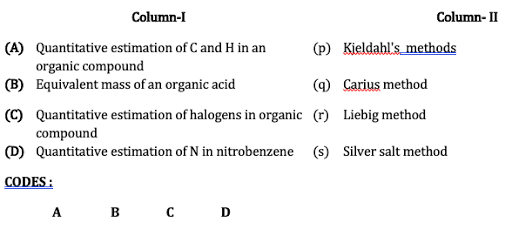•  R s q p
•  p r s q
•  q p r s
•  s q p r
Solution

All are self-explanatory
Q6.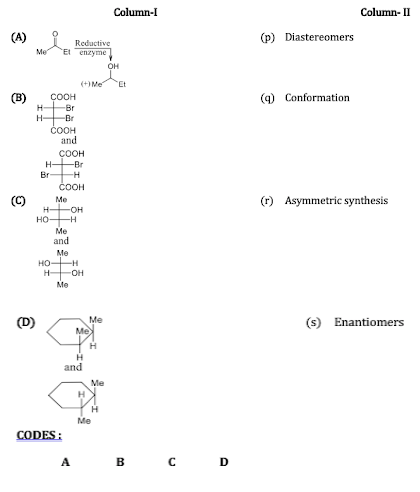•  s p q r
•  r s p q
• r q s p
•  r p s p
Solution
(a r) The synthesis of chirals compounds from achiral compounds by the use of chiral reagent is called asymmetric synthesis (b p) They are diastereomers (c s) They are enantiomers (d p) The and isomers (diastereomers) (e q) Skew and anti (staggered ) forms are conformational isomers

Q7.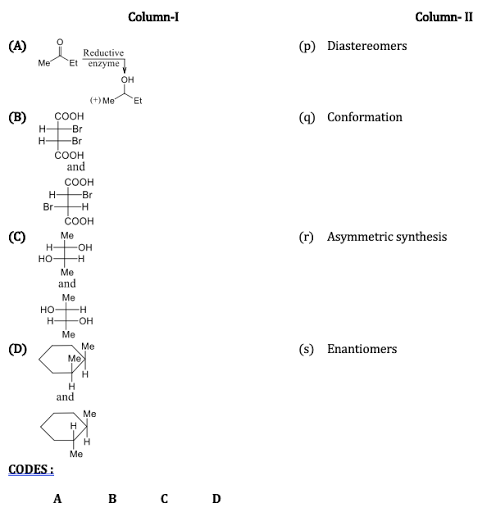•  s p q r
•  r s p q
•  r q s p
•  r p s p
Solution
(a→ r) The synthesis of chirals compounds from achiral compounds by the use of chiral reagent is called asymmetric synthesis (b→ p) They are diastereomers (c→ s) They are enantiomers (d→ p) The cis and trans isomers (diastereomers) (e→ q) Skew and anti (staggered ) forms are conformational isomers

Q8.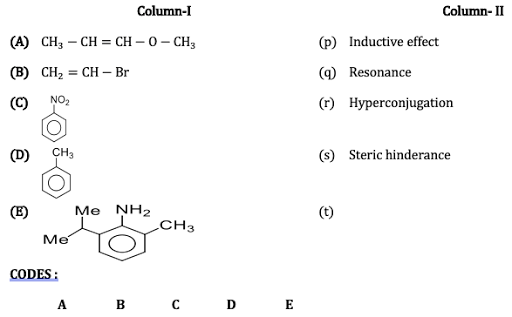•  P,q,r p,q,r,s p,q,r p,q
•  p,q,r p,q p,q p,q,r
•  p,q,r,s p,q,r p,q p,q,r
•  p,q p,q,r p,q p,q,r,s
Solution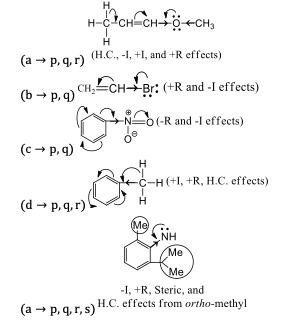Q9.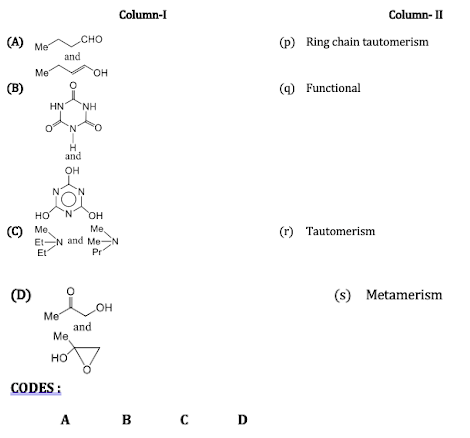•  Q,r q,r s p
•  q,r s p q,r
•  p q,r q,r s
•  q,r q,r p s
Solution

(a q,r) Both functional and tautomerism (b q,r) Both functional and tautomerism (c s) amines to amines are metamers (d p) Ring chain tautomerism
Q10.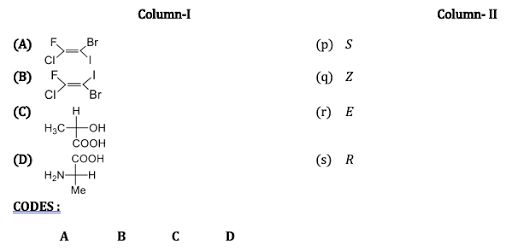•  r q s p
•  s p q r
•  q r s p
• s p r q
Solution
(a q) ( ); two higher priority groups Cl and I are on the same side (b r) ( ); Two higher priority groups Cl and I are on the opposite sides (c s) ( ); priority order: H is on the vertical line; sequence is clockwise and hence ( ) configuration (d p) ( ); priority order : H is on the horizontal line, with one interchange of H and Me; configuration is clockwise, so ( ) configuration (odd number of interchanges)#### Written by: AUTHORNAME

AUTHORDESCRIPTION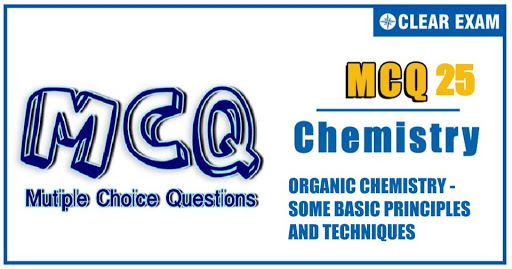## Want to know more

Please fill in the details below:

## Latest NEET Articles\$type=three\$c=3\$author=hide\$comment=hide\$rm=hide\$date=hide\$snippet=hide

Name

ltr
item
BEST NEET COACHING CENTER | BEST IIT JEE COACHING INSTITUTE | BEST NEET & IIT JEE COACHING: ORGANIC CHEMISTRY - SOME BASIC PRINCIPLES AND TECHNIQUES Quiz-25
ORGANIC CHEMISTRY - SOME BASIC PRINCIPLES AND TECHNIQUES Quiz-25